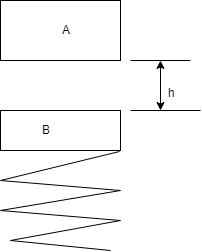# Block A having a mass of 2 kg,is release from rest,fall a distance h = 0.5 m, and strike a plate...

## Question:

Block A having a mass of 2 kg,is release from rest,fall a distance h = 0.5 m, and strike a plate B having a mass of 3 kg. spring stiffness is 100N/m. Determine the maximum deformation of spring.## Spring Stiffness:

When a spring is loaded by some force (compressive or tensile), deflection is observed in the spring due to that force. So, the material of spring opposes that deflection and this opposition is known as spring stiffness. It depends on the force applied and the material of spring.

## Answer and Explanation: 1

Become a Study.com member to unlock this answer! Create your account

Given data:

• Mass of block A is: {eq}{m_A} = 2\;{\rm{kg}} {/eq}
• Mass of block B is: {eq}{m_B} = 3\;{\rm{kg}} {/eq}
• Distance by which block A strikes...

See full answer below.

#### Learn more about this topic:Hooke's Law & the Spring Constant: Definition & Equation

from

Chapter 4 / Lesson 19
202K

After watching this video, you will be able to explain what Hooke's Law is and use the equation for Hooke's Law to solve problems. A short quiz will follow.